# Dividing Fractions - A Guide## Dividing Fractions

Hi there. In this math guide, I cover the topic of dividing fractions.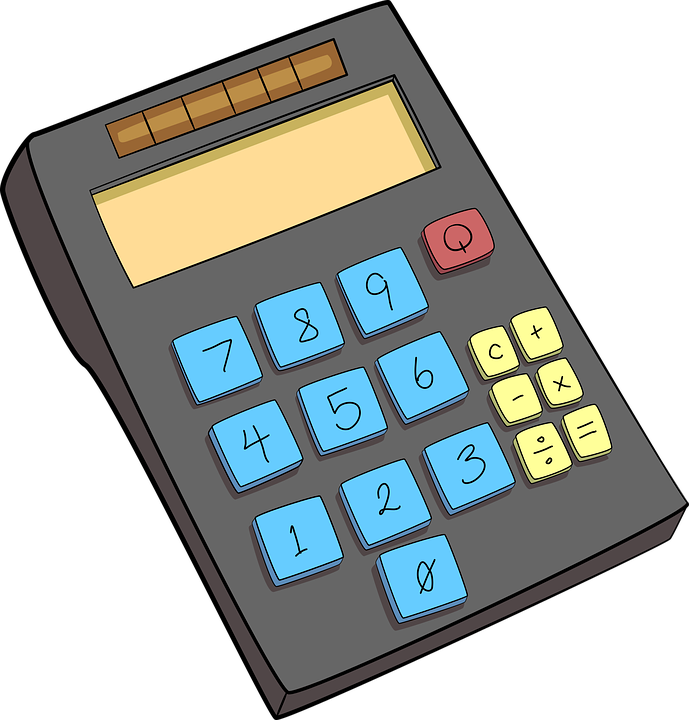Pixabay Image Source

Screenshots made with Windows Snipping Tool, witeboard.com & Wacom Tablet & Stylus Pen.

## Review Of Multiplying Fractions

As dividing fractions is a bit of an extension of multiplying fractions, it is a good idea to review multiplying fractions first.

Assuming that the denominators (bottom numbers in fractions) are not zero, we can multiply two fractions in the following way.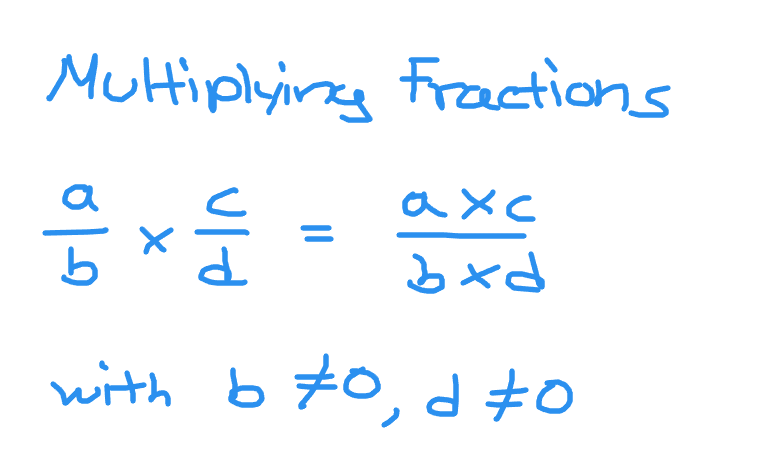## Dividing Fractions With Examples

Dividing fractions contains an extra step before multiplying fractions. When it comes to dividing two fractions you have to flip the second fraction and then change the division sign in the middle into multiplication. You then have two fractions being multiplied. Screenshot image below.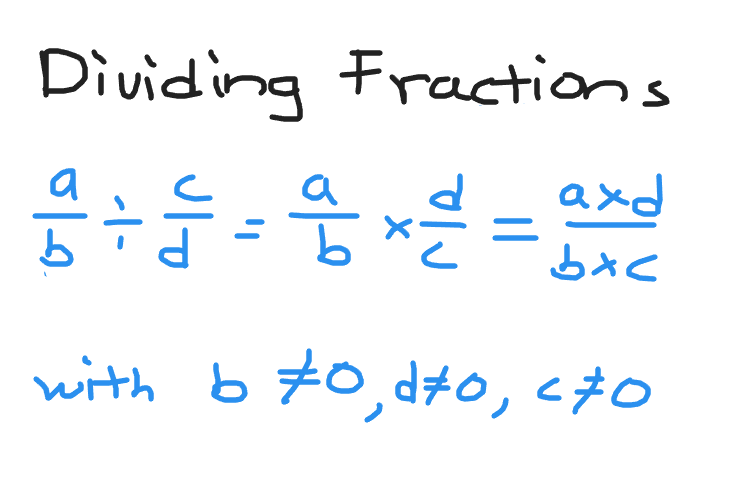The bottom numbers (denominators) cannot be zero plus the numerator of the second fraction cannot be zero. We avoid division by zero.

Example One

Evaluate one half divided by three tenths.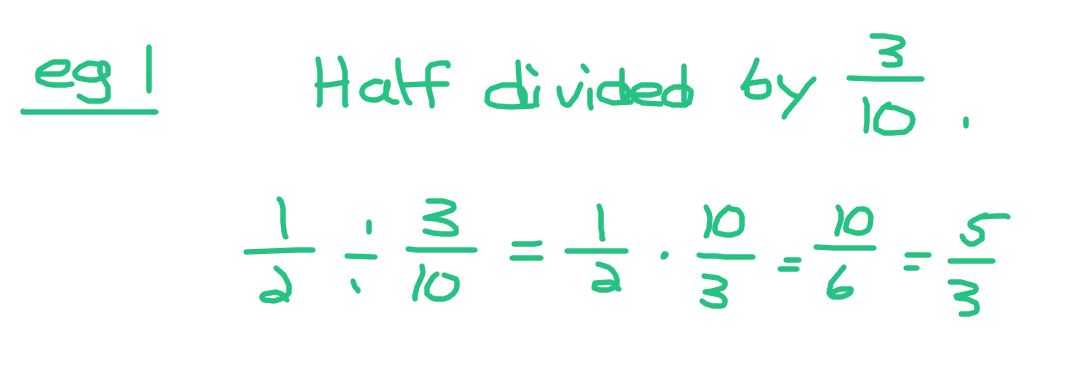Example Two

Divide three fifths by nine sevenths.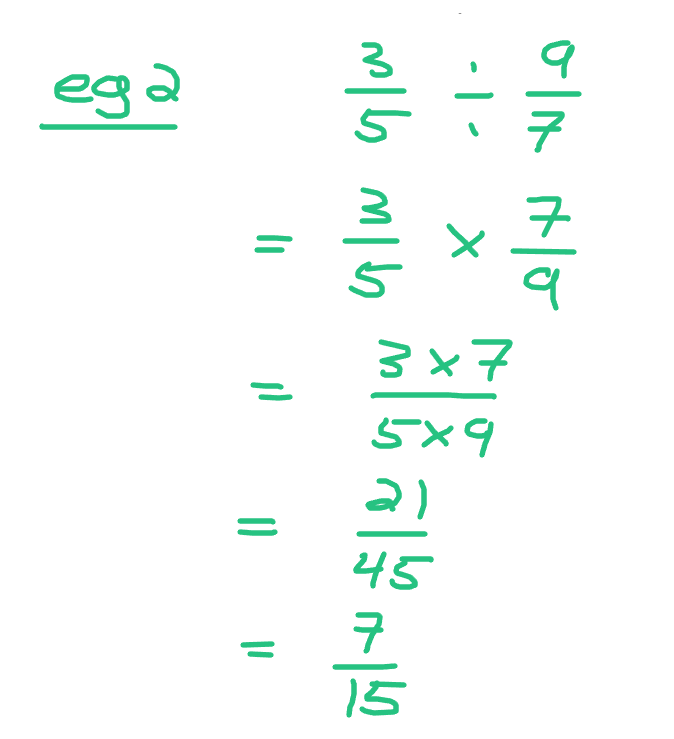Example Three

Jerry has ten cakes. He wants each slice to be one-eighth of a cake. How many slices would there be if Jerry cuts the cakes accordingly?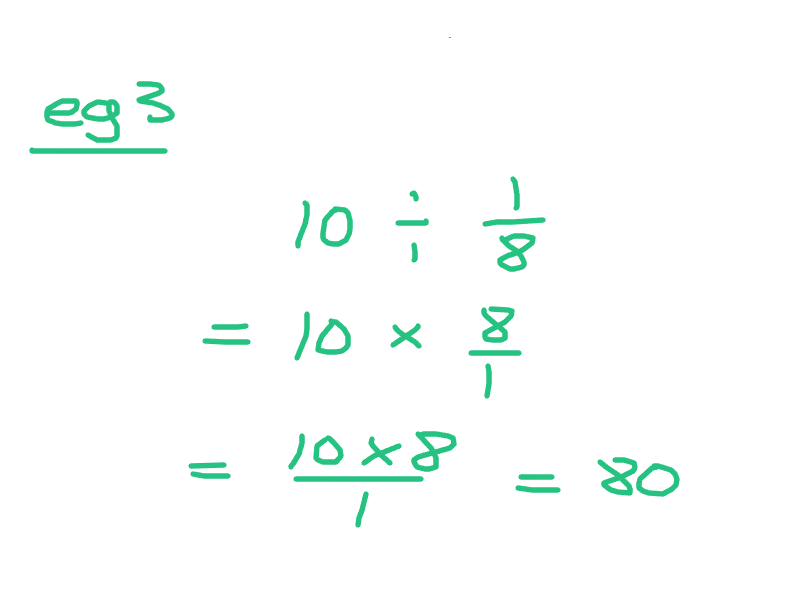Example Four - Mixed Numbers Case

There may be a rare case where you divide mixed numbers together. You have to convert the mixed numbers to improper fractions. Once you have the improper fractions divide the fractions as usual.

Divide five and a quarter by two and a third.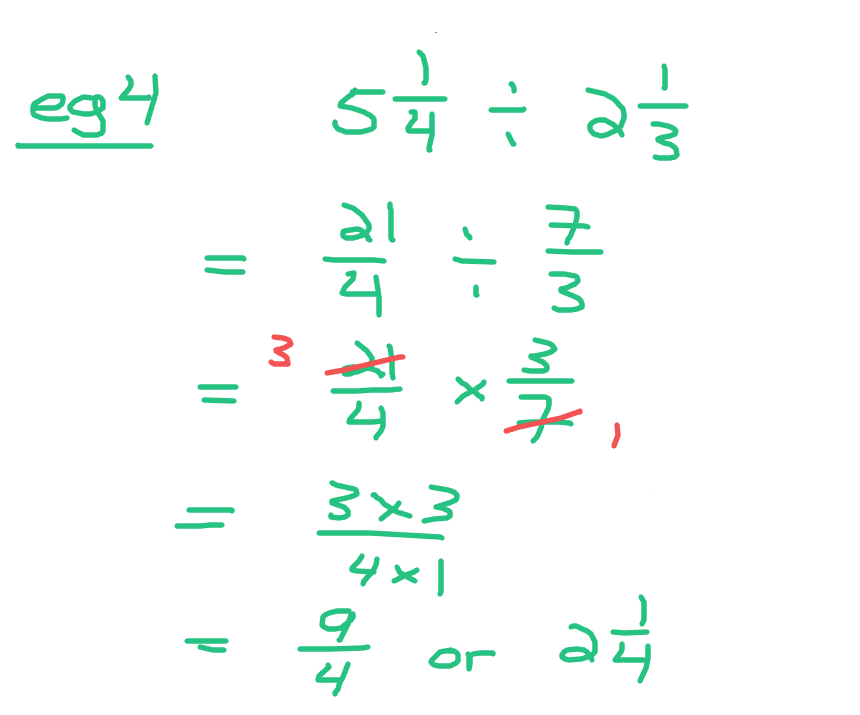Posted with STEMGeeks

0
0
0.000